# 1.4 An introductory tour

This section provides an overview of the wide range of tasks for which Pyxplot can be used. Detailed explanations of the syntax of Pyxplot commands will follow in later chapters, but most of the examples here will work if entered directly at a Pyxplot command prompt.

The mathematical environment

Pyxplot’s mathematical environment comes with many standard functions built-in. To see a list of them1, type

show functions


The show command is Pyxplot’s interactive documentation system; to obtain a list of things that can be shown type

show


Pyxplot is an object-orientated language, and its built-in functions live in a module called defaults. Its members may be listed by printing the module object.

print defaults


Taking as an example the built-in function log10(x), it can be evaluated as in almost any other programming language. The defaults module is special in that its functions are always accessible to the user’s namespace:

pyxplot> print log10(5)
0.69897

This function returns numerical data, but Pyxplot has other data types too. For example, the primeFactors function returns a list:

pyxplot> print primeFactors(1001)
[7, 11, 13]

The rgb(r,g,b) function returns a color object, which can be used in Pyxplot’s vector graphics commands:

pyxplot> print rgb(1,1,0)
rgb(1,1,0)

and the time.fromUnix(t) function returns a date object from a Unix time:

pyxplot> print time.fromUnix(946684800)
Sat 2000 Jan 1 00:00:00 UTC

Many commonly-used physical constants are built into Pyxplot’s physics module, phy. For example, the speed of light:

pyxplot> print phy.c
299792.46 km/s

Numbers in Pyxplot have physical units, and hence the speed of light is displayed in km/s. If you would rather know how many miles light travels in a year, you can change the display unit, here making use of the fact that the variable ans is always set to the result of the last calculation:

pyxplot> print phy.c
299792.46 km/s
pyxplot> set unit preferred miles/year
pyxplot> print ans
5.87849967e+12 mi/yr

It is easy to use Pyxplot as a desktop calculator to solve many problems which would conventionally need careful conversion between physical units. For example:

• What is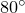F in Celsius?

pyxplot> print 80*unit(oF) / unit(oC)
26.666667

• How long does it take for light to travel from the Sun to the Earth?2

pyxplot> print unit(AU) / phy.c
499.00478 s

• What wavelength of light corresponds to the ionisation energy of hydrogen (13.6 eV)?3

pyxplot> print phy.c * phy.h / (13.6 * unit(eV))
91.164844 nm

• What is the escape velocity of the Earth?4

pyxplot> print sqrt(2 * phy.G * unit(Mearth) / unit(Rearth))
11.186605 km/s

Graph plotting

The simplest way to plot a graph in Pyxplot is simply to follow the plot command with the name of a function to be plotted, e.g.:

plot sin(x)


If a data file is to be plotted, its filename is put in place of a named function. In this example the fifth column of a data file is plotted against the second, including only those data points where the fourth column is larger than two:

plot 'data.dat' using 2:5 select $4>2  In the example below, three Bessel functions are plotted over specified horizontal and vertical ranges: plot [0:10][-0.5:1] besselJ(0,x), besselJ(1,x), besselJ(2,x)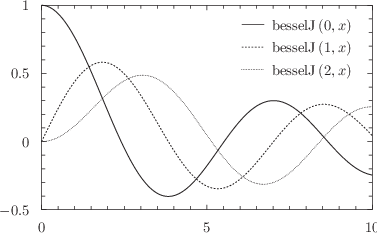With a little additional configuration, it is possible to produce three-dimensional plots like this (this example is taken from Section 8.14.1; see Example 8.14.1):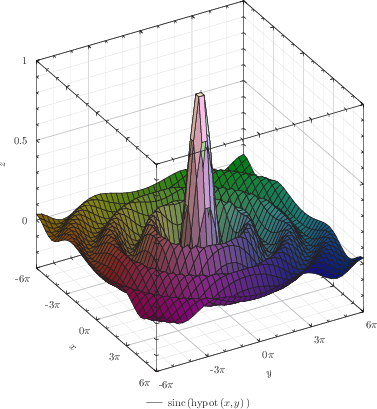It is also possible to produce colormaps with custom color scales; this is documented in full in Section 8.12. Pyxplot includes functions for converting wavelengths of light into colors; they are used here to create a color map of the electromagnetic spectrum: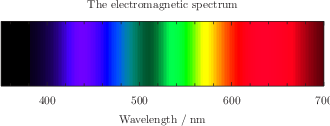This pair of images demonstrates RGB color mixing (see Example 8.12.1):Generating data tables Pyxplot can output tables of data to disk, using a similar syntax to that used for plotting graphs. The data can either be sampled from functions, or read in from another data file: tabulate tan(x)  A common application of the tabulate command is filter or re-format the contents of data files. For example, the command below takes only the third and seventh columns out of a data file, and converts the latter from degrees into radians: tabulate 'data.dat' using 3:$7*unit(deg)/unit(rad)


The same effect could be achieved by setting radians as the default unit of angle:

set unit of angle radians
tabulate 'data.dat' using 3:\$7*unit(deg)


More sophisticated data processing is also possible; this example produces a histogram of the values in the fourth column of a datafile, and then outputs that histogram as a new data file:

histogram h() 'data.dat' using 4
tabulate h(x)


Solving equations

Pyxplot can solve systems of equations numerically; the following example evaluates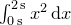:

pyxplot> print int_dx(x**2,0*unit(s),2*unit(s))
2.6666667 s**3

The solve command can be used to solve systems of simultaneous equations, such as this system with two variables:

pyxplot> solve x+y=1 , 2*x+3*y=7 via x,y
pyxplot> print "x=%s; y=%s"%(x,y)
x=-4; y=5

The minimise and maximise commands find the extrema of functions; here they are used to find the minimum of the function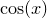closest to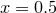:

pyxplot> x=0.5
pyxplot> minimise cos(x) via x
pyxplot> print x
3.1415927

All of the examples shown so far have used only real numbers, but Pyxplot can also perform algebra on complex numbers. By default, evaluation of sqrt(-1) throws an error – the emergence of complex numbers is often an indication that a calculation has gone wrong – but complex arithmetic can be enabled by typing

pyxplot> set numerics complex
pyxplot> print sqrt(-1)
i

Many of the mathematical functions which are built into Pyxplot can take complex arguments, for example

pyxplot> set numerics complex
pyxplot> print exp(2+3*i)
(-7.3151101+1.0427437i)
pyxplot> print sin(i)
1.1752012i
pyxplot> print arg(2+3*i)
0.98279372
pyxplot> print Re(2+3*i)
2

Vector graphics

Pyxplot’s graph-plotting canvas can also be used for drawing general vector graphics, using simple commands such as:

box from -8,-4 to 8,4 with fillcolor green
text "Pyxplot" at 2,3
arrow from 0,0 to -4,2
line from -5,0 to 5,0


These commands are described in detail in Chapter 10. They interface neatly to the vector data type in Pyxplot’s mathematical environment, to ease geometric construction. Thus, it is quite possible for mathematically-minded users to multiply transformation matrices with position vectors on the graphics canvas to calculate where objects should be drawn. The following example uses a rotation matrix to draw a big arrow at angleto the vertical:

rotate(a) = matrix( [[cos(a),-sin(a)], \
[sin(a), cos(a)] ] )
pos = vector(0,5)*unit(cm)
theta = 30*unit(deg)
arrow from 0,0 to rotate(theta)*pos with linewidth 3


Footnotes